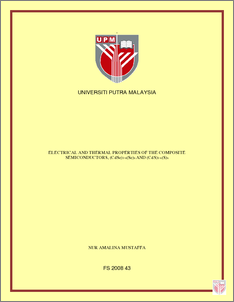# Electrical And Thermal Properties Of Thecomposite Semiconductors, (Cdse)1-X(Se)X And (Cds)1-X(S)X

## Citation

Mustaffa, Nur Amalina (2008) Electrical And Thermal Properties Of Thecomposite Semiconductors, (Cdse)1-X(Se)X And (Cds)1-X(S)X. Masters thesis, Universiti Putra Malaysia.

## Abstract

A series of (CdSe)1-x(Se)x and (CdS)1-x(S)x composite semiconductors were prepared with different stoichiometric compositions of Se and S with x = 0 to x = 0.8 both in the interval of 0.2 by varying the ratio of CdSe:Se and CdS:S in a reaction mixture. The following powder of CdSe, Se and CdS, S were used as the starting materials. X-ray diffraction analysis was carried out in order to investigate the structural character of the composites obtained. For both samples, analysis of the X-ray diffractogram revealed that the samples were in hexagonal form. Atomic Force Microscopy (AFM) was used for analyzing the surface morphology of the composites samples. Parallel plate method was used to determine the dc conductivity of all samples in the temperature range of 300 – 460 K. Both samples, (CdSe)1-x(Se)x and (CdS)1-x(S)x , show variation in ln σ with 1000/T (K-1) that indicated that there are three distinct temperature zones with three different characteristic regions. This behaviour suggests that two types of conduction mechanisms were presence. The first region is identified as the extrinsic region, the second region is the intermediate region and the third region is the intrinsic region. For both series of samples, the conductivity obtained for all series of samples shown similar trend, the dc conductivity increased as the temperature increased. For (CdSe)1-x(Se)x composite where x = 0, the dc conductivity at 300 K is about 10-8 S/cm and increase up to 10-5 S/cm at 460 K. While for (CdS)1-x(S)x composite where x = 0, the dc conductivity at 300 K is about 10-10 S/cm and increase up to 10-8 S/cm at 503 K. The activation energies were calculated from the Arrhenius relation and the values of the activation energy indicated that all the prepared samples were semiconductors. The ac conductivity properties of polycrystalline (CdSe)1-x(Se)x and (CdS)1-x(S)x were studied in temperature range of 300 – 523 K and frequency range of 100 Hz – 1 MHz using Impedance Analyzer. Obtained data of ac conductivity revealed that at low frequency σAC (ω) was independent of frequency and proportional to ωs at higher frequency for all samples. The values of the frequency exponent, s were found to decrease with increasing temperature which suggested that the dominant transport process as Correlated Barrier Hopping (CBH). Thermal diffusivity values of both (CdSe)1-x(Se)x and (CdS)1-x(S)x were investigated using photoflash technique. Thermal diffusivity value decrease as we increase the Se and S compositions in the samples.Preview
PDF
FS_2008_43a.pdfView Item